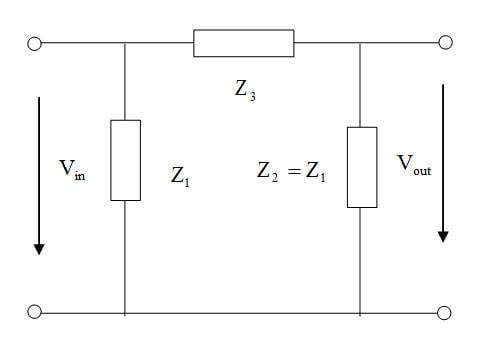# Two-port network models

## Homework Statement

Design a π section symmetrical attenuator to provide a voltage attenuation of 15 dB and a characteristic impedance of 600 Ω.Zc=600Ω,$\alpha$=15dB (attenuation)
Z1,Z3=?

## The Attempt at a Solution

$\alpha$[dB]=20log(Vin/Vout)=15
$\alpha$=5.623

Vin/Vout=(Z1+Z2)/Z1

Zc=√ZocZsc
Zoc=(Z1(Z1+Z3))/(2Z1+Z3)
Zsc=(Z1Z3)/(Z1+Z3)

Zc=Z1√1-2/(2+Z3/Z1)

Z1D=600

Z3/Z1=4.623
D=0.835

Z1=718Ω
Z3=3320Ω

Last edited by a moderator:

## Answers and Replies

NascentOxygen
Staff Emeritus
Science Advisor
I'd be inclined to show the arrows for Vin and Vout upside down to how you have, though I guess it makes no difference.

Vin/Vout=(Z1+Z2)/Z1 should be Z3 not Z2

Your figures give -15 dB, and 609.6 ohms for Zin

I have made a typing error,it is Z3